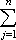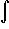#Interactive Real Analysis

Next | Previous | Glossary | Map

## 7.4. Lebesgue Integral

### Example 7.4.6(c): Lebesgue Integral for Bounded Functions

Is the Dirichlet function restricted to [0, 1] Lebesgue integrable? If so, find the integral.
The Dirichlet function restricted to [0, 1] is a simple function and we have already seen that its integral is zero. But we have now two definitions of the integral in case of a simple function, and we need to show that both definitions agree.

If f(x) =cj XEj is a simple function, then the infimum over the integrals of all simple functions bigger than f must be smaller than the integral of f, which is itself a simple function. In other words, if f is a simple function than we have automatically

I*(f)Lf(x) dx =cj m(Ej)
Similarly we have
I*(f)Lf(x) dx =cj m(Ej)
But since I*(f)LI*(f)L we have for every simple function f thatcj m(Ej)I*(f)LI*(f)Lcj m(Ej)
so that for simple functions both definitions of the Lebesgue integral agree. In particular, the Lebesgue integral of the Dirichlet function over [0, 1] is then zero.
Next | Previous | Glossary | Map